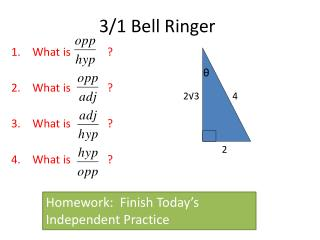DownloadDownload Presentation3/1 Bell Ringer

# 3/1 Bell Ringer

Download Presentation## 3/1 Bell Ringer

- - - - - - - - - - - - - - - - - - - - - - - - - - - E N D - - - - - - - - - - - - - - - - - - - - - - - - - - -
##### Presentation Transcript

1. θ 3/1 Bell Ringer 2√3 4 What is ? What is ? What is ? What is ? 2 Homework: Finish Today’s Independent Practice

2. 3/1 News and Notes • Perfection Award: 4thPeriod • Tests back • Reminder about test corrections • QUIZ FRIDAY!

3. Bell Ringer Review θ 2√3 4 2

4. New Material • All we’re doing today is naming the functions that represent the ratios from the bell ringer!

5. SOH-CAH-TOA 5 3 Θ 4

6. 5 Now fill in with regard to 3 Θ 4

7. Quick Check - SOHCAHTOA • What trig ratio is represented by 3/5 in following diagram? • 3 = adj • 5 = hyp • Adj/hyp = COSINE!  cos(Θ) = 3/5 Θ 5 3 4

8. Guided Practice • A painter needs to reach the top of a 15 foot tall sign. He uses a ladder of length x to reach the top of the sign. The angle formed where the ladder meets the ground should be labeled Θ. • Step 1: DRAW A PICTURE, labeling what you can.

9. Step 2: What trig ratio would you use to represent this situation? Explain why. Sin because opp = 15 and hyp = x. • Step 3: Write the full trig equation • HONORS: If Θ = 60°, what is the length of the ladder?

10. Independent Practice • We will finish with an Exit Ticket!

11. ACT Question of the Day In the figure below, what is ? A. sine of angle G only B. cosine of angle G only C. cosine of angle H only • sine of angle G and cosine of angle H. • Sine of angle H and cosine of angle G. Homework: Finish IP